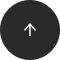SQL Server

# How to Get the Current Date and Time (Without Time Zone) in T-SQL

#### Operators:

GETDATE(), CURRENT_TIMESTAMP, SYSDATETIME()

#### Problem:

You’d like to get the current date and time in T-SQL, but you don’t want the time zone offset.

#### Solution:

We’ll use GETDATE(), CURRENT_TIMESTAMP, and SYSDATETIME() to get the current date and time without the time zone offset. The first two functions allow us to get the current time with a lower precision. (GETDATE() is a T-SQL function, while CURRENT_TIMESTAMP is a SQL Standard function; both functions return the same data type). The third function, SYSDATETIME(), gets a higher-precision time.

```SELECT CURRENT_TIMESTAMP ;
```

Here’s the result of the query:

`2019-08-14 10:01:06.983`

#### Discussion:

In a SQL Server database, the `CURRENT_TIMESTAMP` function returns the current date and time (i.e. the time on the machine where that SQL Server instance runs) as a `datetime` datatype. `Datetime` is a lower-precision time that has a maximum of three fractional seconds. (In our example, this is 983.)

GETDATE() is similar to the `CURRENT_TIMESTAMP` and returns the same result. The difference is that `CURRENT_TIMESTAMP` is SQL standard, while GETDATE() is specific to SQL Server.

```SELECT GETDATE() ;
```

Of course, if you want to get a higher-precision current date and time without a time zone offset, you can use the SYSDATETIME() function. This function returns a `datetime2` data type with seven fractional seconds. Look at the example below:

```SELECT SYSDATETIME() ;
```

Here’s the result:

`2019-08-14 10:01:06.9754163`

#### Recommended articles: